# Bricks II

3/4 bricks weighs 6 kg and 2/3 bricks. How weighs one whole brick?

x =  72 kg

### Step-by-step explanation:

3/4x = 6+ 2/3 x

3/4•x = 6+ 2/3•x

x = 72

Our simple equation calculator calculates it.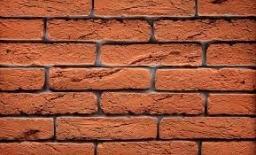Did you find an error or inaccuracy? Feel free to write us. Thank you!Tips to related online calculators
Do you have a linear equation or system of equations and looking for its solution? Or do you have a quadratic equation?

## Related math problems and questions:

• Brick weightThe brick weighs 2 kg and a half bricks. How much does one brick weigh?
• Two bricks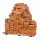One brick weighs 1kg and half a brick, how many weigh two bricks?
• Bag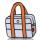Nelly found interesting point. An empty bag weighs 4 kg less than full. And empty bag is 5 times easier than full. How many kg weigh things in this bag?
• Bob and Bobek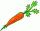Bobek has 2 cabbages and 5 carrots, Bob has 2 cabbages and 9 carrots. When Bobek stepped on the scale weight 5800 g and when Bob weight 8960 g. Actual Bob weight is 8 kg and Bobek 5 kg. How much weighs one carrot?
• Apples 5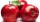In six crates are 45 kg of apples. In five crates were the same amount and in one crate was 3 kg of apples more. How many kg of apples were in each crate?
• Brick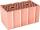Brick weight before drying is 5.3 kg. After drying, the bricks is 1 kg lighter and after firing is 0.7 kg lighter than after drying. What is the weight of the brick after drying and firing?
• A shopkeeper 3A shopkeeper sells 8 1/3 kg, 10 1/4 kg and 11 1/5 kg of apples on 3 consecutive days. What is the total weight of apples sold?
• Can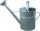Watering can full of water weighs 10 kg. Half-full can weighs 5.5 kg. How much weigh can?
• Center of gravityThe mass points are distributed in space as follows - specify by coordinates and weight. Find the center of gravity of the mass points system: A1 [1; -20; 3] m1 = 46 kg A2 [-20; 2; 9] m2 = 81 kg A3 [9
• Cooks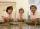Four cooks cleaned 5 kg of potatoes for 10 minutes. How many cook would have to work clean 9 kg of potatoes for 12 minutes?
• Brick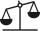Isosceles scale has on one side all brick and second weight 1 kg and 1/4 of brick. The balance is in equilibrium. What is the weight of a brick?
• Packets weight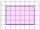The weight of three packets are 3 2/5 kg + 2 1/3 kg + 5 1/2 kg. Find total weight of all the three packets.
• Volunteers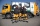Volunteers helped clean up 8.2 kg of trash in one neighborhood and 11 1/2 kg in another. They sent 2 3/5 kg to be recycled and threw the rest away. How many kilograms of trash did they throw away?
• BricksOpenings in perforated bricks occupy 10% and brick has dimensions 30 cm, 15 cm and 7.5 cm. Calculate a) the weight of a perforated bricks, if you know that the density of the full brick material is p = 1800 kg/m3 (1.8 kg/dm3) b) the number of perforated
• Milk2cheese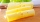From 40 liters of milk is produced 8 kg of cheese how many liters of milk are needed to produce 2 kg of cheese?
• Fractions and mixed numerals(a) Convert the following mixed numbers to improper fractions. i. 3 5/8 ii. 7 7/6 (b) Convert the following improper fraction to a mixed number. i. 13/4 ii. 78/5 (c) Simplify these fractions to their lowest terms. i. 36/42 ii. 27/45 2. evaluate the follow
• Children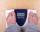Margaret and Zdena weigh the same and Petra 3 kg more. Together weigh 156 kg. How much do they weigh?